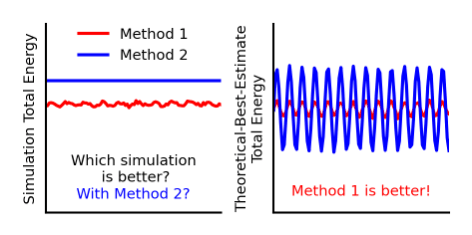# Energy-conserving molecular dynamics is not energy conserving!Molecular dynamics simulations are widely used to study molecules and materials and lots of effort is put into making these simulations obey the physical laws. Energy conservation law is obviously one of the most important laws that MD should respect. Once you have run the simulations and see the coveted flat total energy line, should you be happy that it is energy-conserving? What does it mean, ‘energy-conserving’ anyway? In our thought-provoking article we argue that such ‘energy-conserving’ MD simulations actually are not energy-conserving and stricter and clearer definitions are very helpful while choosing and designing better MD algorithms. We suggest error measures to estimate the deviation from ‘true energy conservation’ that can be easily adopted for the robust evaluation of the quality of MD as we demonstrate with our MD implementation in MLatom@XACS. In addition, these error measures are universal enough to be applied even for MDs from the novel 4D-spacetime AI models which directly predict trajectory without calculating forces and potential energies.

Ensuring that MD stays energy-conserving during the simulation in the NVE ensemble is typically important for increasing the robustness and preventing the trajectory from, e.g., unphysical heating up. The problem is that the total energy is evaluated using the approximate potential with which the MD was propagated in the first place. For the geometries where the approximate potential underestimates the potential energy, MD leads to higher velocities and kinetic energy at the next time step which eventually lead to higher frequencies of motions and even to unphysical dissociation of a molecule. Thus, even when the total energy is not changing during the MD propagation, the trajectory still may be very wrong.

We have the paradox: the conservation of total energy is important and… not important?!

We suggest to resolve this absurd paradox by adopting very simple terminology and error measures. First we need to realize that the conservation of energy is indeed important, but conservation of the exact energy, not the total energy calculated during the simulations with the approximate potential. Thus, we should term MD with constant approximate total energy as simulation-energy conserving. What we want to achieve is then true-energy conservation, when the exact total energy is conserving.

If we look at trajectories from the perspective of how much they deviate from the true-energy conservation, everything falls nicely in place. While we usually have no access to the exact energy, we can estimate deviation from it by calculating the theoretical-best estimate (TBE) of the total energy during the MD propagation. Such TBEs can be conveniently calculated for the sample of the trajectory points by evaluating the single-point potential energies with the more accurate method and adding them to the kinetic energy:

Single-point calculations for a subset of points is often an affordable solution when it is impossible to run MD with more expensive TBE potential. Such TBE total energies are basically never conserving and better reflect the quality of the trajectory. In our above example, when the approximate potential underestimate the potential energy, the TBE total energy will be systematically too high during MD which indicates that the system unphysically gains energy from the vacuum and nicely explain the higher frequencies and dissociations. The degree of deviation can be very simply evaluated as:

We demonstrate the utility of the TBE total energy estimates to explain the too high-frequency IR spectra and show how this error measure can be used to reliably choose the method for MD propagation to obtain the good IR spectrum. In addition, we show that simulation-energy non-conserving MD can be of better quality than simulation-energy conserving MD which can be easily confirmed with the TBE analysis.

All results were obtained with our new implementation of MD with both machine learning potentials and quantum mechanical methods in open-source MLatom. The simulations can be also run on MLatom@XACS cloud.

Paper:

This site uses Akismet to reduce spam. Learn how your comment data is processed.